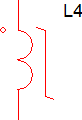# PWL Inductors

The SIMPLIS Piecewise Linear (PWL) inductor is used to model a current dependent inductance, such as a saturating transformer. The PWL inductor is defined with a series of points on an x-y plane where the flux linkages are on the vertical axis and the inductor current is on the horizontal axis. With this definition, the flux is always continuous as the current through the inductor varies.A good description of the PWL modeling techniques using PWL inductors can be found in the Piecewise Linear Inductors section of SIMPLIS is Always Piecewise Linear, All the Time.

## Circuit Example

The PWL Transformers topic describes the use of a PWL inductor in a transformer application.